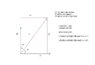# Orthonormal Bases: Determining Coefficients for Arbitrary Vector

• mrxtothaz

#### mrxtothaz

If we have a vector that can be expressed in terms of some finite list of basis elements. If we have an orthonormal basis for a vector space V, then a vector v can be expressed as <v,e1>e1 +...+ <v,en>en. This appears to be widely used for many results (such as Gram-Schmidt), but the motivation for this is not clear to me. Not only that, I don't understand why this is the case (geometrically).

Obtaining the coefficients for a given vector (in terms of an orthonormal basis) using the inner product of the vector v with each basis element... why does this work?

If you consider the version of the dot product involving cosine, $v\cdot e_{1}=|v||e_{1}|cos\theta=|v|cos\theta$ because $e_{1}$ is a unit vector. This is just the "$e_1$ component" of v (rather than the x or y component of v).
I included a poorly done illustration in Paint.

#### Attachments

•Orthonormal Basis.JPG
18.2 KB · Views: 531
It works because it works on basis vectors. Define the linear operator A by
$$Av = \sum_{i=1}^n \langle v, e_i \rangle e_i.$$
Then for any k,
$$Ae_k = \sum_{i=1}^n \langle e_k, e_i \rangle e_i = \sum_{i=1}^n \delta_{ki} e_i = e_k.$$
It follows that A = I, so Av = v for any v.